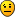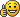## How to write an acceleration equation in thyme?

If you have problems installing or running Algodoo, have found a bug or need in-game help - this is it!

### How to write an acceleration equation in thyme?

Is there any possible way to write a code to determine the acceleration of an object ( both horizontal acceleration and vertical acceleration separately ) ?faytree

Posts: 942
Joined: Mon Nov 05, 2012 1:37 pm
Location: uhhh Jakku

### Re: How to write an acceleration equation in thyme?

Thats totally simple tho and should be asked in the thyme subforum. Just declare a variable _prevVel = vel (using the black mini console of your object), and then calculate the accelleration using poststep:

accell = (vel-_prevVel)/e.dt;
_prevVel = vel;

The entire accelleration would be math.vec.len(accell) while the components would be accell(0) and accell(1)
FRA32

Posts: 213
Joined: Wed Dec 03, 2014 9:51 pm

### Re: How to write an acceleration equation in thyme?

FRA32 wrote:Thats totally simple tho and should be asked in the thyme subforum. Just declare a variable _prevVel = vel (using the black mini console of your object), and then calculate the accelleration using poststep:

accell = (vel-_prevVel)/e.dt;
_prevVel = vel;

The entire accelleration would be math.vec.len(accell) while the components would be accell(0) and accell(1)

Thanks a lot, your method is much simpler and effective comparing to other methodsfaytree

Posts: 942
Joined: Mon Nov 05, 2012 1:37 pm
Location: uhhh Jakku